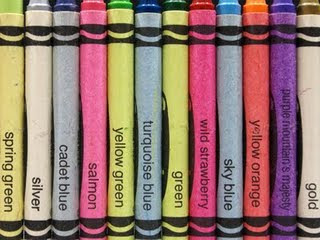## Tuesday, October 20, 2009

### Abby's Bob Ratio 10.20.09

BOB1GREETINGS!! (:

PART TO PART RATIO'S

1. - related to blue to related to green
- 3: 3
- 3 to 3
- rtb/ rtg = 3/3
- 1.0: 1
- 100 % :100
- Im not sure if theres an equivalent ratio for this one..

2. - 1 word to 2 or more words
- 1: 2
- 1 to 2
- word/ words = 1/2
- 0.5: 1
- 50% :100
- 1/2 = 12/24 ( I multiply the numerator and the denominator by twelve )

3. - vowels to consonants
- 43: 77
- 43 to 77
- v/ c = 43/ 77
- 0.55: 1
- 55.8 % :100
- 43/ 77 = 86/ 154 ( I multiply the numerator and the denominator by 2 )

PART TO WHOLE RATIO

1. - blue's to total
- 3: 12
- 3 to 12
- b's/ t = 3/12
- 0.25= 1
- 25% =100
- 3/12 = 1/4 ( I divided the numerator and the denominator by 3 )

2. - a to all vowels
- 6: 43
- 6 to 43
- a/ all vowels = 6/43
- 0.13= 1
- 13. 9 % = 100
- 6/43= 18/ 129 ( I multiply the numerator and the denominator by 3 )

THREE PART RATIO

1. - gold , green's to blue's
- 4: 3
- 4 to 3
- gold, green's/ blue's = 4/3
-1.33 (continuous) : 1
- 133.3 % : 100
- 4/3 = 8/6 ( I multiply the numerator and the denominator by 2 )

2. - green's , silver to total
- 4: 12
- 4 to 12
- green's, silver/ total = 4/12
- 0.33 ( continuous ) : 1
- 33.3 % : 100
- 4/12 = 1/3 ( I divide the numerator and the denominator by 4 )

QUESTIONS:

1. Fractions,decimals and percents are like a chain. Tell me why or why not? Explain and show your answer.

2. How do you make a fraction for a three part ratio?

3. If you were ask to make another part to part ratio about this picture besides for the one I have, what would it be? Tell me the ratio notation, fraction, decimal, percent and its equivallent ratio.

PLEASE ANSWER THE FOLLOWING QUESTIONS AT THE COMMENT BOX!! (:
THANKS FOR READING MY POST! GOOD LUCK FOR THE TEST TOMORROW!! HOPE YOU'LL DO GOOD!! (:
AND DONT FORGET TO COMMENT!! (:

Byeee!! (:

BOB2
This is my bob for extra marks. I almost failed my test so that I need to work on some stuff. Like when to use a ratio table, I have no idea when to use a ratio table ( that's embarrassing ). Like the last question.

18) In a student election Carol gets 60% of the votes. If there were 485 votes cast, how many votes did Carol get?
My answer is 194 instead of 291. When I was doing the test, I have no clue what to do until when we went over it in class. I didn't know that we have to use a ratio table to show our work. What I did was I used a calculator. I kinda guess.

The other mistakes I got is kind of like the same thing as the one above because they all use ratio table to find the answers..

Question:
1. How do you know when to use a ratio table?

Eric.P 8-73

Good job Abby.
1. Fractions can be divided to make decimals. decimals can be multiplied to make percent.
2.iunno It'd be nice if u could explain that on a comment.
3.silver to total 1:12 1/12 0.08 8% 2:24

Caitlin873

GOOD JOB ABBY!

1.) well a fraction is made by the ratio notation (i think),soo then to make a decimal jus divide the numerator and the denominator of the fraction, then to get the percent get the decimal and multiply it by 100.

2.) uhh... for this question i dont kno . maybe you cant make a fraction for a three term ratio but if it can be done on a two term ratio i could work on a three term ratio.

3.)uhh... im not sure if im right.
turquoise blue to total 1:12 1/12 0.08 8% .

Michael8-73

Bob2
1.When the question has anything to do with complicated percentages.